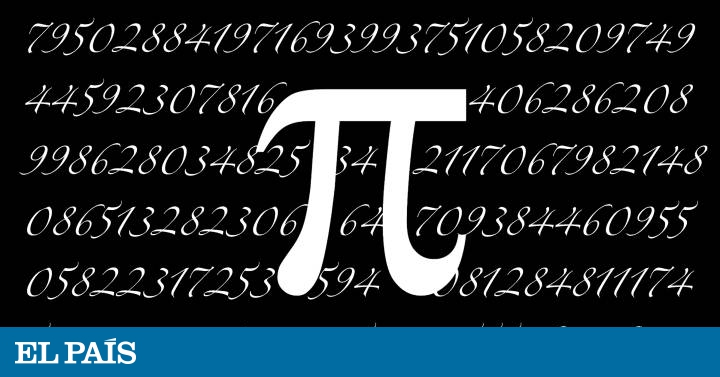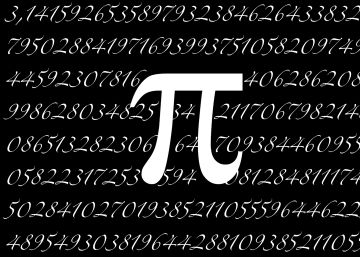Mon. Nov 18th, 2019

# Is 3.14 a good approximation of Pi? | ScienceIf you ask the value of the number Pi To anyone, there will be some who will answer that it is 3.14. But nevertheless, as many others know, it is not true. Pi it has infinite decimal figures not periodic, not just two, that is, it is an irrational number. However, for some things it is very useful to be able to approximate irrational, such as Pi, by rational numbers, with which it is easier to work. A rational number is one that can be expressed as a fraction, that is, a quotient of two integers, called numerator (the top part) and denominator (the bottom part). To approximate Pi, the rational number 3.14 is usually used, that is 157/50, But is this a good rounding? How can we say, in general, that a rational approach is?

A first criterion is that it is close to the value of the irrational number. Without other data, we could say that 3.1416 is a better approximation than 3.14. Another criterion, often contradictory to the above, is that it be a simple expression. Sometimes both properties occur: for example, although fractions 157/50 and 22/7 are both approximations of Pi, the second is simpler and is also closer to the real value of Pi: there is no doubt which to choose.

There is a dichotomy generated by the choice of the error term: if its size is large enough, all numbers can be represented, but if it is too small, none can be represented

In general, when an irrational number is approximated by a fraction, the “price to be paid” is the size of the denominator and the “profit” is the “goodness” of the approximation, that is, the size of the error. The objective is to optimize the choices of the denominator and the error. We can also establish that these two values ​​are related to each other (for example the error can be inversely proportional to the square of the chosen denominator). This allows to have, at the same time, high precision and simplicity in the representation. In 1837 the mathematician Gustav Lejeune Dirichlet He showed that there are infinite different ways to approximate irrational numbers with fractions, admitting precisely an error term inversely proportional to the square of the denominator.

But the problem was not solved here, but quite the opposite, new questions appeared: is it possible to look for expressions that introduce a smaller error term? Are there numbers that can be represented with restrictions (error and denominator) stronger than others? Which? Richard Duffin and Albert Schaeffer studied this problem in 1941. They observed that there is a dichotomy generated by the choice of the error term: if its size is large enough, all numbers can be represented, but if it is too small, none can be represented. They technically formulated this idea but were not able to prove it, so that the problem remained open for decades. As happens many times in mathematics, and in particular in number theory, a simple question to explain is very difficult to solve.

This summer, Dimitri Koukoulopoulos of the University of Montreal and James Maynard of the University of Oxford came up with the answer. Both are very reputable researchers in the call analytical number theory, which uses analysis techniques to obtain arithmetic results, but they are not experts in the particular area of diophantine approach, which encompasses the problem, which made its announcement especially surprising. It was at a congress of number theory that took place in July this year in Cetaro, Italy, before a hundred experts from the field. Article, On the Duffin-Schaefer conjecture It is an extensive work that introduces numerous innovative mathematical tools, and is still under review by scientific journals. Although the mathematical community will only fully accept the demonstration of the result after a very detailed analysis by experts, numerous researchers have already echoed what appears to be a new milestone.

There are infinite different ways to approximate irrational numbers with fractions, admitting precisely an error term inversely proportional to the square of the denominator

Daniele Casazza he is a postdoctoral researcher in the ICMAT

Agate Timón García-Longoria He is responsible for Communication and Disclosure in the ICMAT

It is a section dedicated to mathematics and the environment in which they are created, coordinated by the Institute of Mathematical Sciences (ICMAT), in which researchers and members of the center describe the latest advances in this discipline, share meeting points between mathematics and other social and cultural expressions and remember those who marked their development and knew how to transform coffee into theorems. The name evokes the definition of the Hungarian mathematician Alfred Rényi: "A mathematician is a machine that transforms coffee into theorems."

Editing and coordination: Agate Timon (ICMAT).